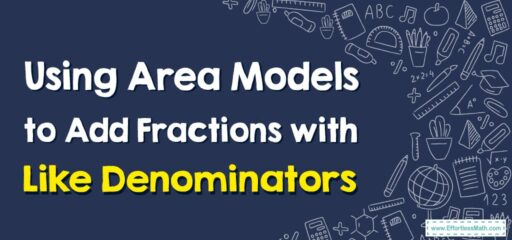# How to Use Area Models to Add Fractions with Like Denominators

Area models provide a visual way to represent and add fractions with like denominators.## A step-by-step guide to Using Area Models to Add Fractions with Like Denominators

### Step 1: Understand the concept of an area model

An area model is a visual representation of a mathematical concept using a grid, rectangle, or square. Each section of the model represents a part of the whole, and the entire model represents the total value.

### Step 2: Create a combined area model for both fractions

Since the fractions have like denominators, you can create a single area model to represent both fractions. Draw a rectangle and divide it into equal parts based on the denominator of the fractions.

Example: To add $$\frac{2}{5}$$ and $$\frac{3}{5}$$:

• Draw a rectangle and divide it into 5 equal parts for the denominator 5.

### Step 3: Shade the parts representing each fraction

Shade the number of parts indicated by the numerator of each fraction.

• In the example, shade 2 parts to represent $$\frac{2}{5}$$ and 3 more parts to represent $$\frac{3}{5}$$.

### Step 4: Add the fractions using the area model

Combine the shaded parts of the area model to represent the sum of the fractions.

• For the example above, the shaded parts from the two fractions ($$\frac{2}{5}$$ and $$\frac{3}{5}$$) represent the sum of the fractions.

### Step 5: Write the sum as a fraction

Count the total number of shaded parts and write the sum as a fraction with the same denominator as the original fractions.

• In the example above, there are a total of 5 shaded parts, so the sum is $$\frac{5}{5}$$.

### Step 6: Simplify the fraction, if necessary

If the fraction can be simplified, do so to express the sum in its simplest form.

• In this example, $$\frac{5}{5}$$ can be simplified to 1.

So, using an area model, we find that $$\frac{2}{5} + \frac{3}{5} = 1$$. This method helps to visually demonstrate the addition of fractions with like denominators, making it more accessible and easier to comprehend.

### What people say about "How to Use Area Models to Add Fractions with Like Denominators - Effortless Math: We Help Students Learn to LOVE Mathematics"?

No one replied yet.

X
30% OFF

Limited time only!

Save Over 30%

SAVE $5 It was$16.99 now it is \$11.99